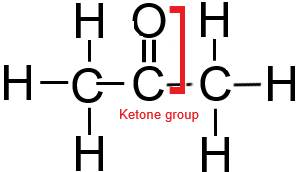# Sigma and pi bonds Calculator

Sigma and pi bonds Calculator

Sigma and pi bond calculator is a simple online tool to count the number of sigma and pi bonds present in a molecule with the help of using the type of bonds(single, double, and triple) present in a molecule.

## How to use Sigma and pi bond calculator?

All you need to count the number of single, double, and triple bonds present in a molecule.

 Determine the type of the bond (Single, Double, or Triple bond). All single bonds are sigma bonds. (Single bond = 1 sigma bond).A double bond contains a sigma bond and a pi bond. (Double bond = 1 sigma + 1 pi bond).The triple bond contains one sigma bond and two pi bonds. (Triple bond = 1 sigma + 2 pi bonds).For example – If the molecule has 3 single bonds and 1 double bond, that means, we have 4 sigma bonds and 1 pi bond. [∴ 3 single bond means 3 sigma bond and 1 double bond means 1 sigma + 1 pi bond].

So, to get the number of sigma and pi bonds in a molecule, just count the single, double, and triple bonds present in the molecule, and then press calculate to evaluate the sigma and pi bonds present in a molecule.

## How to calculate the sigma and pi bonds?

### How many sigma and pi bonds are in Ethyne (C2H2)?• By looking at the above structure, we see, Ethyne contains 2 single bonds and 1 triple bond.
• 2 single bond means 2 sigma bonds, and one triple bond means 1 sigma + 2 pi bond.
• Therefore, in ethyne (C2H2), a total of 3 sigmas and 2 pi bonds are present.Or use a sigma and pi bond calculator. In ethyne structure, the number of single bonds is 2, the double bond is zero, and the triple bond is 1.### How many sigma and bonds are present in benzene (C6H6)?

As we learned earlier, all double bonds contain one pi bond and one sigma bond.

In benzene, we notice that there are 3 double bonds, so undoubtedly we have 3 pi bonds + 3 sigma bonds. Also, we see, 9 single bonds also here, so 9 sigma bonds. So when we add we get 12 sigma and 3 pi bonds in Benzene.So, in Benzene (C6H6), the number of sigma bonds is 12, and the pi bond is 3.

 Count the sigma and pi bonds in Acetone (CH3COCH3)According to the above figure, there are eight single bonds and one double bond in CH3COCH3.8 single bond means 8 sigma bond and 1 double means (1 sigma + 1 pi bond).So, in acetone (CH3COCH3), the number of sigma bonds is 9 and the pi bond is only 1.Check the detailed guide –

## FAQ

### How to use the Sigma and pi bond calculator tool?

To use the Sigma and pi bond calculator tool, you must have the data given below-

• The number of single bonds.
• The number of double bonds.
• The number of triple bonds.

After that, fill the data in respective fields and hit the calculate button of the Sigma and pi bonds calculator to get the answer.Let’s say, a molecule does not have any double bonds, then either leave the field of “Number of double bonds” blank or fill in 0.

### What are sigma and pi bonds, and How you can calculate them?

The Sigma bond is represented by (σ). It is the only bond in single covalent bonds. A Sigma bond is formed when two atoms share electrons and their valence levels directly overlap (end to end).

The pi bond is represented by (𝛑) and it arises from the overlapping of parallel p orbitals. The pi bond is found in multiple covalent bonds i.e. double covalent bonds which consist of one sigma bond and one pi bond, and triple covalent bonds which consist of one sigma bond and two pi bonds.

To count the sigma and pi bonds, all you need to remember is that, (1 single bond = 1 sigma bond), (1 double bond = 1 sigma bond + 1 pi bond), (1 triple bond = 1 sigma bond + 2 pi bonds).

Did you like it?### Vishal Goyal

My name is Vishal Goyal and I am the founder of Topblogtenz. I hold a degree in B.tech (Chemical Engineering) and have a strong passion for the life sciences and chemistry. As a highly qualified and experienced chemistry tutor with 4 years of experience, I possess a deep understanding of the unique challenges that students often encounter when attempting self-study in the field of chemistry. I have created this website as a comprehensive resource for those who are seeking guidance and support in their chemistry studies. I have brought together a team of experts, including experienced researchers, professors, and educators, to provide our readers with accurate and engaging information on a wide range of chemistry and science topics. Our goal is to make complex subjects like chemistry accessible and understandable for all. I hope you find the information and resources on our site helpful in your studies. Let's connect through LinkedIn: https://www.linkedin.com/in/vishal-goyal-2926a122b/

Share it...

## Connect with us

Email = [email protected]